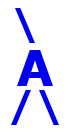## AIRES library reference: getxmaxdata.

```c
c     Routine directly callable from C/C++ (it could be necessary to
c     append an underscore, "_", to its name depending on the C/C++
c     compiler used).
c
c
subroutine getxmaxdata(vsl, xmaxdata, nmaxdata, nshowers)
c
c     Returning shower maximum and related data.
c
c     Written by: S. J. Sciutto, La Plata 2003.
c
c
c     Arguments:
c     =========
c
c     vsl............. (input, integer) Integer switch to select
c                      between vertical (vsl = 1) and slant (vsl = 2)
c                      depths. Any other value of vsl is equivalent to
c                      vsl = 1.
c     xmaxdata........ (output, double precision, array(5)) Array
c                      containing information about the depth of shower
c                      maximum:
c                         xmaxdata(1) --- Average Xmax.
c                         xmaxdata(2) --- RMS error of the mean.
c                         xmaxdata(3) --- Standard deviation.
c                         xmaxdata(4) --- Minimum Xmax.
c                         xmaxdata(5) --- Maximum Xmax.
c     nmaxdata........ (output, double precision, array(5)) Array
c                      containing information about the number of
c                      charged particles at maximum:
c                         nmaxdata(1) --- Average Xmax.
c                         nmaxdata(2) --- RMS error of the mean.
c                         nmaxdata(3) --- Standard deviation.
c                         nmaxdata(4) --- Minimum Xmax.
c                         nmaxdata(5) --- Maximum Xmax.
c     nshowers........ (output, integer) Number of showers in the data
c                      set (Showers with converged fits). If nshowers
c                      is less or equal than 0, then the arrays
c                      xmaxdata and nmaxdata are undefined.
c
c
c<--->
```

 These pages are maintained by Sergio J. Sciutto Back to AIRES library index Back to AIRES home